Physics > Electricity and MagnetismShowing results 21 to 30 of 30, on page 3 of 4
 DESCRIPTION EQUATION Number density of charge carriers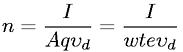Ohm's Law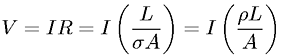Potential due to a line charge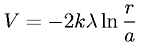Poynting vector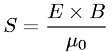Resistance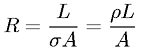Self inductance of a solenoid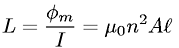Torque on a current loop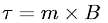Voltage equation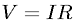Wave equation for magnetic field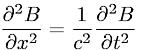Yukawa Potential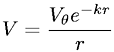Go to page: 1  2  3